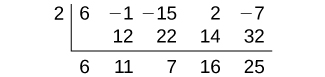5.5 Zeros of polynomial functions

 Page 1 / 14
In this section, you will:
• Evaluate a polynomial using the Remainder Theorem.
• Use the Factor Theorem to solve a polynomial equation.
• Use the Rational Zero Theorem to find rational zeros.
• Find zeros of a polynomial function.
• Use the Linear Factorization Theorem to find polynomials with given zeros.
• Use Descartes’ Rule of Signs.
• Solve real-world applications of polynomial equations

A new bakery offers decorated sheet cakes for children’s birthday parties and other special occasions. The bakery wants the volume of a small cake to be 351 cubic inches. The cake is in the shape of a rectangular solid. They want the length of the cake to be four inches longer than the width of the cake and the height of the cake to be one-third of the width. What should the dimensions of the cake pan be?

This problem can be solved by writing a cubic function and solving a cubic equation for the volume of the cake. In this section, we will discuss a variety of tools for writing polynomial functions and solving polynomial equations.

Evaluating a polynomial using the remainder theorem

In the last section, we learned how to divide polynomials. We can now use polynomial division to evaluate polynomials using the Remainder Theorem    . If the polynomial is divided by $\text{\hspace{0.17em}}x–k,\text{\hspace{0.17em}}$ the remainder may be found quickly by evaluating the polynomial function at $\text{\hspace{0.17em}}k,\text{\hspace{0.17em}}$ that is, $\text{\hspace{0.17em}}f\left(k\right)\text{\hspace{0.17em}}$ Let’s walk through the proof of the theorem.

Recall that the Division Algorithm    states that, given a polynomial dividend $\text{\hspace{0.17em}}f\left(x\right)\text{\hspace{0.17em}}$ and a non-zero polynomial divisor $\text{\hspace{0.17em}}d\left(x\right)\text{\hspace{0.17em}}$ where the degree of $\text{\hspace{0.17em}}\text{\hspace{0.17em}}d\left(x\right)\text{\hspace{0.17em}}$ is less than or equal to the degree of $\text{\hspace{0.17em}}f\left(x\right)$ , there exist unique polynomials $\text{\hspace{0.17em}}q\left(x\right)\text{\hspace{0.17em}}$ and $\text{\hspace{0.17em}}r\left(x\right)\text{\hspace{0.17em}}$ such that

$\text{\hspace{0.17em}}f\left(x\right)=d\left(x\right)q\left(x\right)+r\left(x\right)$

If the divisor, $\text{\hspace{0.17em}}d\left(x\right),\text{\hspace{0.17em}}$ is $\text{\hspace{0.17em}}x-k,\text{\hspace{0.17em}}$ this takes the form

$f\left(x\right)=\left(x-k\right)q\left(x\right)+r$

Since the divisor $\text{\hspace{0.17em}}x-k\text{\hspace{0.17em}}$ is linear, the remainder will be a constant, $\text{\hspace{0.17em}}r.\text{\hspace{0.17em}}$ And, if we evaluate this for $\text{\hspace{0.17em}}x=k,\text{\hspace{0.17em}}$ we have

$\begin{array}{ccc}\hfill f\left(k\right)& =& \left(k-k\right)q\left(k\right)+r\hfill \\ & =& 0\cdot q\left(k\right)+r\hfill \\ & =& r\hfill \end{array}$

In other words, $\text{\hspace{0.17em}}f\left(k\right)\text{\hspace{0.17em}}$ is the remainder obtained by dividing $\text{\hspace{0.17em}}f\left(x\right)\text{\hspace{0.17em}}$ by $\text{\hspace{0.17em}}x-k.\text{\hspace{0.17em}}$

The remainder theorem

If a polynomial $\text{\hspace{0.17em}}f\left(x\right)\text{\hspace{0.17em}}$ is divided by $\text{\hspace{0.17em}}x-k,\text{\hspace{0.17em}}$ then the remainder is the value $\text{\hspace{0.17em}}f\left(k\right).\text{\hspace{0.17em}}$

Given a polynomial function $\text{\hspace{0.17em}}f,$ evaluate $\text{\hspace{0.17em}}f\left(x\right)\text{\hspace{0.17em}}$ at $\text{\hspace{0.17em}}x=k\text{\hspace{0.17em}}$ using the Remainder Theorem.

1. Use synthetic division to divide the polynomial by $\text{\hspace{0.17em}}x-k.\text{\hspace{0.17em}}$
2. The remainder is the value $\text{\hspace{0.17em}}f\left(k\right).\text{\hspace{0.17em}}$

Using the remainder theorem to evaluate a polynomial

Use the Remainder Theorem to evaluate $\text{\hspace{0.17em}}f\left(x\right)=6{x}^{4}-{x}^{3}-15{x}^{2}+2x-7\text{\hspace{0.17em}}$ at $\text{\hspace{0.17em}}x=2.\text{\hspace{0.17em}}$

To find the remainder using the Remainder Theorem, use synthetic division to divide the polynomial by $\text{\hspace{0.17em}}x-2.\text{\hspace{0.17em}}$The remainder is 25. Therefore, $\text{\hspace{0.17em}}f\left(2\right)=25.\text{\hspace{0.17em}}$

Use the Remainder Theorem to evaluate $\text{\hspace{0.17em}}f\left(x\right)=2{x}^{5}-3{x}^{4}-9{x}^{3}+8{x}^{2}+2\text{\hspace{0.17em}}$ at $\text{\hspace{0.17em}}x=-3.\text{\hspace{0.17em}}$

$\text{\hspace{0.17em}}f\left(-3\right)=-412\text{\hspace{0.17em}}$

Using the factor theorem to solve a polynomial equation

The Factor Theorem is another theorem that helps us analyze polynomial equations. It tells us how the zeros of a polynomial are related to the factors. Recall that the Division Algorithm.

$f\left(x\right)=\left(x-k\right)q\left(x\right)+r$

If $\text{\hspace{0.17em}}k\text{\hspace{0.17em}}$ is a zero, then the remainder $\text{\hspace{0.17em}}r\text{\hspace{0.17em}}$ is $\text{\hspace{0.17em}}f\left(k\right)=0\text{\hspace{0.17em}}$ and $\text{\hspace{0.17em}}f\left(x\right)=\left(x-k\right)q\left(x\right)+0\text{\hspace{0.17em}}$ or $\text{\hspace{0.17em}}f\left(x\right)=\left(x-k\right)q\left(x\right).\text{\hspace{0.17em}}$

Notice, written in this form, $\text{\hspace{0.17em}}x-k\text{\hspace{0.17em}}$ is a factor of $\text{\hspace{0.17em}}f\left(x\right).\text{\hspace{0.17em}}$ We can conclude if $\text{\hspace{0.17em}}k\text{\hspace{0.17em}}$ is a zero of $\text{\hspace{0.17em}}f\left(x\right),\text{\hspace{0.17em}}$ then $\text{\hspace{0.17em}}x-k\text{\hspace{0.17em}}$ is a factor of $f\left(x\right).\text{\hspace{0.17em}}$

A laser rangefinder is locked on a comet approaching Earth. The distance g(x), in kilometers, of the comet after x days, for x in the interval 0 to 30 days, is given by g(x)=250,000csc(π30x). Graph g(x) on the interval [0, 35]. Evaluate g(5)  and interpret the information. What is the minimum distance between the comet and Earth? When does this occur? To which constant in the equation does this correspond? Find and discuss the meaning of any vertical asymptotes.
The sequence is {1,-1,1-1.....} has
how can we solve this problem
Sin(A+B) = sinBcosA+cosBsinA
Prove it
Eseka
Eseka
hi
Joel
June needs 45 gallons of punch. 2 different coolers. Bigger cooler is 5 times as large as smaller cooler. How many gallons in each cooler?
7.5 and 37.5
Nando
find the sum of 28th term of the AP 3+10+17+---------
I think you should say "28 terms" instead of "28th term"
Vedant
the 28th term is 175
Nando
192
Kenneth
if sequence sn is a such that sn>0 for all n and lim sn=0than prove that lim (s1 s2............ sn) ke hole power n =n
write down the polynomial function with root 1/3,2,-3 with solution
if A and B are subspaces of V prove that (A+B)/B=A/(A-B)
write down the value of each of the following in surd form a)cos(-65°) b)sin(-180°)c)tan(225°)d)tan(135°)
Prove that (sinA/1-cosA - 1-cosA/sinA) (cosA/1-sinA - 1-sinA/cosA) = 4
what is the answer to dividing negative index
In a triangle ABC prove that. (b+c)cosA+(c+a)cosB+(a+b)cisC=a+b+c.
give me the waec 2019 questionsByBy